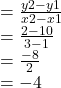## Find the equation of the straight line that passes through the points (1, 10) and (3, 2) ANSWER ASAP

Question

Find the equation of the straight line that passes through the points (1, 10) and (3, 2)

in progress 0
2 weeks 2021-08-31T23:28:07+00:00 2 Answers 0 views 0

2y + 8x = 28

Step-by-step explanation:

Hi there

So we are going to solve this together

m = y – y1/y2- y1 =x – x1/x2 – x1

y – 10/ 2- 10 =x – 1/ 3 -1

y -10/-8 =x – 1/2

cross multiply

2(y – 10) =-8(x -1)

2y – 20= -8x + 8

Collect terms

2y +8x = 8 +20

ANSWER = 2y + 8x = 28

Hope this helps you!

Bye , have a nice day 🙂

y = -4x + 14

Step-by-step explanation:

using the slope intercept form of a line

y = mx + c

Given points

• 1, 10
• 3, 2

slope (m) =taking one point as for filling x and y and finding the y intercept (c)

y =mx + c

10 = (-4) × 1 + c

10 + 4 = c

14 = c

therefore the equation is

y = -4x + 14